x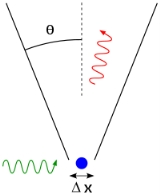Uncertainty principleOverview

In quantum mechanics
Quantum mechanics
Quantum mechanics, also known as quantum physics or quantum theory, is a branch of physics providing a mathematical description of much of the dual particle-like and wave-like behavior and interactions of energy and matter. It departs from classical mechanics primarily at the atomic and subatomic...

, the Heisenberg uncertainty principle states a fundamental limit on the accuracy with which certain pairs of physical properties of a particle, such as position
Position
Position may refer to:* Position , a player role within a team* Position , the orientation of a baby prior to birth* Position , a mathematical identification of relative location...

and momentum
Momentum
In classical mechanics, linear momentum or translational momentum is the product of the mass and velocity of an object...

, can be simultaneously known. In other words, the more precisely one property is measured, the less precisely the other can be controlled, determined, or known.

In his Nobel Laureate speech, Max Born
Max Born
Max Born was a German-born physicist and mathematician who was instrumental in the development of quantum mechanics. He also made contributions to solid-state physics and optics and supervised the work of a number of notable physicists in the 1920s and 30s...

said:
To measure space coordinates and instants of time, rigid measuring rods and clocks are required.Unanswered QuestionsWhy rutherford model didnt work,why it fail?What role the spectroscopy help bohr come up with his atomic modelEncyclopedia
In quantum mechanics
Quantum mechanics
Quantum mechanics, also known as quantum physics or quantum theory, is a branch of physics providing a mathematical description of much of the dual particle-like and wave-like behavior and interactions of energy and matter. It departs from classical mechanics primarily at the atomic and subatomic...

, the Heisenberg uncertainty principle states a fundamental limit on the accuracy with which certain pairs of physical properties of a particle, such as position
Position
Position may refer to:* Position , a player role within a team* Position , the orientation of a baby prior to birth* Position , a mathematical identification of relative location...

and momentum
Momentum
In classical mechanics, linear momentum or translational momentum is the product of the mass and velocity of an object...

, can be simultaneously known. In other words, the more precisely one property is measured, the less precisely the other can be controlled, determined, or known.

In his Nobel Laureate speech, Max Born
Max Born
Max Born was a German-born physicist and mathematician who was instrumental in the development of quantum mechanics. He also made contributions to solid-state physics and optics and supervised the work of a number of notable physicists in the 1920s and 30s...

said:
To measure space coordinates and instants of time, rigid measuring rods and clocks are required. On the other hand, to measure momenta and energies, devices are necessary with movable parts to absorb the impact of the test object and to indicate the size of its momentum. Paying regard to the fact that quantum mechanics is competent for dealing with the interaction of object and apparatus, it is seen that no arrangement is possible that will fulfill both requirements simultaneously."

Published by Werner Heisenberg
Werner Heisenberg
Werner Karl Heisenberg was a German theoretical physicist who made foundational contributions to quantum mechanics and is best known for asserting the uncertainty principle of quantum theory...

in 1927, the uncertainty principle was a key discovery in the early development of quantum theory
Quantum theory
Quantum theory may mean:In science:*Quantum mechanics: a subset of quantum physics explaining the physical behaviours at atomic and sub-atomic levels*Old quantum theory under the Bohr model...

. It implies that it is impossible to simultaneously measure the present position while also determining the future motion of a particle, or of any system small enough to require quantum mechanical treatment. Intuitively, the principle can be understood by considering a typical measurement of a particle. It is impossible to determine both momentum and position by means of the same measurement, as indicated by Born above. Assume that its initial momentum has been accurately calculated by measuring its mass, the force applied to it, and the length of time it was subjected to that force. Then to measure its position after it is no longer being accelerated would require another measurement to be done by scattering light or other particles off of it. But each such interaction will alter its momentum by an unknown and indeterminable increment, degrading our knowledge of its momentum while augmenting our knowledge of its position. So Heisenberg argues that every measurement destroys part of our knowledge of the system that was obtained by previous measurements.The uncertainty principle states a fundamental property of quantum systems, and is not a statement about the observational success of current technology.

The principle states specifically that the product of the uncertainties in position and momentum is always equal to or greater than one half of the reduced Planck constant ħ, which is defined as the re-scaling h/(2π) of the Planck constant
Planck constant
The Planck constant , also called Planck's constant, is a physical constant reflecting the sizes of energy quanta in quantum mechanics. It is named after Max Planck, one of the founders of quantum theory, who discovered it in 1899...

h. Mathematically, the uncertainty relation between position and momentum arises because the expressions of the wavefunction in the two corresponding bases
Basis (linear algebra)
In linear algebra, a basis is a set of linearly independent vectors that, in a linear combination, can represent every vector in a given vector space or free module, or, more simply put, which define a "coordinate system"...

are Fourier transforms of one another (i.e., position and momentum are conjugate variables
Conjugate variables
Conjugate variables are pairs of variables mathematically defined in such a way that they become Fourier transform duals of one-another, or more generally are related through Pontryagin duality. The duality relations lead naturally to an uncertainty in physics called the Heisenberg uncertainty...

). In the mathematical formulation of quantum mechanics
Mathematical formulation of quantum mechanics
The mathematical formulations of quantum mechanics are those mathematical formalisms that permit a rigorous description of quantum mechanics. Such are distinguished from mathematical formalisms for theories developed prior to the early 1900s by the use of abstract mathematical structures, such as...

, any non-commuting
Commutator
In mathematics, the commutator gives an indication of the extent to which a certain binary operation fails to be commutative. There are different definitions used in group theory and ring theory.-Group theory:...

operators are subject to similar uncertainty limits.

## Historical introduction

Werner Heisenberg
Werner Heisenberg
Werner Karl Heisenberg was a German theoretical physicist who made foundational contributions to quantum mechanics and is best known for asserting the uncertainty principle of quantum theory...

formulated the Uncertainty Principle at Niels Bohr
Niels Bohr
Niels Henrik David Bohr was a Danish physicist who made foundational contributions to understanding atomic structure and quantum mechanics, for which he received the Nobel Prize in Physics in 1922. Bohr mentored and collaborated with many of the top physicists of the century at his institute in...

's institute in Copenhagen, while working on the mathematical foundations of quantum mechanics.

In 1925, following pioneering work with Hendrik Kramers, Heisenberg developed matrix mechanics
Matrix mechanics
Matrix mechanics is a formulation of quantum mechanics created by Werner Heisenberg, Max Born, and Pascual Jordan in 1925.Matrix mechanics was the first conceptually autonomous and logically consistent formulation of quantum mechanics. It extended the Bohr Model by describing how the quantum jumps...

, which replaced the ad-hoc old quantum theory
Old quantum theory
The old quantum theory was a collection of results from the years 1900–1925 which predate modern quantum mechanics. The theory was never complete or self-consistent, but was a collection of heuristic prescriptions which are now understood to be the first quantum corrections to classical mechanics...

with modern quantum mechanics. The central assumption was that the classical concept of motion does not fit at the quantum level, and that electrons in an atom did not travel on sharply defined orbits. Rather, the motion was smeared out in a strange way: the Fourier transform
Fourier transform
In mathematics, Fourier analysis is a subject area which grew from the study of Fourier series. The subject began with the study of the way general functions may be represented by sums of simpler trigonometric functions...

of time only involving those frequencies that could be seen in quantum jumps.

Heisenberg's paper did not admit any unobservable quantities like the exact position of the electron in an orbit at any time; he only allowed the theorist to talk about the Fourier components of the motion. Since the Fourier components were not defined at the classical frequencies, they could not be used to construct an exact trajectory
Trajectory
A trajectory is the path that a moving object follows through space as a function of time. The object might be a projectile or a satellite, for example. It thus includes the meaning of orbit—the path of a planet, an asteroid or a comet as it travels around a central mass...

, so that the formalism could not answer certain overly precise questions about where the electron was or how fast it was going.

The most striking property of Heisenberg's infinite matrices for the position and momentum is that they do not commute
Commutativity
In mathematics an operation is commutative if changing the order of the operands does not change the end result. It is a fundamental property of many binary operations, and many mathematical proofs depend on it...

. Heisenberg's canonical commutation relation
Canonical commutation relation
In physics, the canonical commutation relation is the relation between canonical conjugate quantities , for example:[x,p_x] = i\hbar...

indicates by how much:(see derivations
Uncertainty principle derivations
There have been several mathematical improvements on the Heisenberg uncertainty principle. Since the time of the original formulation, the uncertainty relation has become increasingly nuanced; our understanding of it has undergone both a qualitative and a quantitative change...

)

and this result did not have a clear physical interpretation in the beginning.

In March 1926, working in Bohr's institute, Heisenberg realized that the non-commutativity
Commutativity
In mathematics an operation is commutative if changing the order of the operands does not change the end result. It is a fundamental property of many binary operations, and many mathematical proofs depend on it...

implies the uncertainty principle. This implication provided a clear physical interpretation for the non-commutativity, and it laid the foundation for what became known as the Copenhagen interpretation
Copenhagen interpretation
The Copenhagen interpretation is one of the earliest and most commonly taught interpretations of quantum mechanics. It holds that quantum mechanics does not yield a description of an objective reality but deals only with probabilities of observing, or measuring, various aspects of energy quanta,...

of quantum mechanics. Heisenberg showed that the commutation relation implies an uncertainty, or in Bohr's language a complementarity
Complementarity (physics)
In physics, complementarity is a basic principle of quantum theory proposed by Niels Bohr, closely identified with the Copenhagen interpretation, and refers to effects such as the wave–particle duality...

. Any two variables that do not commute cannot be measured simultaneously — the more precisely one is known, the less precisely the other can be known. Heisenberg wrote:
It can be expressed in its simplest form as follows: One can never know with perfect accuracy both of those two important factors which determine the movement of one of the smallest particles—its position and its velocity. It is impossible to determine accurately both the position and the direction and speed of a particle at the same instant.

One way to understand the complementarity between position and momentum is by wave-particle duality. If a particle described by a plane wave passes through a narrow slit in a wall like a water-wave passing through a narrow channel, the particle diffracts and its wave comes out in a range of angles. The narrower the slit, the wider the diffracted wave and the greater the uncertainty in momentum afterward. The laws of diffraction require that the spread in angle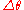is about, where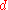is the slit width and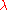is the wavelength. Reasoning based on the de Broglie relation, shows that the size of the slit and the range in momentum of the diffracted wave are related by Heisenberg's rule: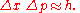In his celebrated 1927 paper, "Über den anschaulichen Inhalt der quantentheoretischen Kinematik und Mechanik" ("On the Perceptual Content of Quantum Theoretical Kinematics and Mechanics"), Heisenberg established this expression as the minimum amount of unavoidable momentum disturbance caused by any position measurement, but he did not give a precise definition for the uncertainties Δx and Δp. Instead, he gave some plausible estimates in each case separately. In his Chicago lecture he refined his principle: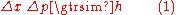But it was Kennard in 1927 who first proved the modern inequality: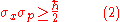where ħ = h/2π, and σx, σp are the standard deviations of position and momentum. Heisenberg himself only proved relation (2) for the special case of Gaussian states. However, it should be noted that σx and Δx are not the same quantities. σx and σp as defined in Kennard are obtained by making repeated measurements of position on an ensemble of systems and by making repeated measurements of momentum on an ensemble of systems and calculating the standard deviation of those measurements. The Kennard expression, therefore, says nothing about the simultaneous measurement of position and momentum.

A rigorous proof of a new inequality for simultaneous measurements in the spirit of Heisenberg and Bohr has been given recently. The measurement process is as follows: Whenever a particle is localized in a finite interval Δx > 0, then the standard deviation of its momentum satisfieswhile the equal sign is given for Cosine states.

### Terminology and translation

Throughout the main body of his original 1927 paper, written in German, Heisenberg used the word "Unbestimmtheit" ("indeterminacy") to describe the basic theoretical principle. Only in the endnote did he switch to the word "Unsicherheit" ("uncertainty"). However, when the English-language version of Heisenberg's textbook, The Physical Principles of the Quantum Theory, was published in 1930, the translation "uncertainty" was used, and it became the more commonly used term in the English language thereafter.

### Heisenberg's microscopeOne way in which Heisenberg originally argued for the uncertainty principle is by using an imaginary microscope as a measuring device. He imagines an experimenter trying to measure the position and momentum of an electron
Electron
The electron is a subatomic particle with a negative elementary electric charge. It has no known components or substructure; in other words, it is generally thought to be an elementary particle. An electron has a mass that is approximately 1/1836 that of the proton...

by shooting a photon
Photon
In physics, a photon is an elementary particle, the quantum of the electromagnetic interaction and the basic unit of light and all other forms of electromagnetic radiation. It is also the force carrier for the electromagnetic force...

at it.

Problem 1 - If the photon has a short wavelength
Wavelength
In physics, the wavelength of a sinusoidal wave is the spatial period of the wave—the distance over which the wave's shape repeats.It is usually determined by considering the distance between consecutive corresponding points of the same phase, such as crests, troughs, or zero crossings, and is a...

, and therefore a large momentum, the position can be measured accurately. But the photon scatters in a random direction, transferring a large and uncertain amount of momentum to the electron. If the photon has a long wavelength
Wavelength
In physics, the wavelength of a sinusoidal wave is the spatial period of the wave—the distance over which the wave's shape repeats.It is usually determined by considering the distance between consecutive corresponding points of the same phase, such as crests, troughs, or zero crossings, and is a...

and low momentum, the collision does not disturb the electron's momentum very much, but the scattering will reveal its position only vaguely.

Problem 2 - If a large aperture
Aperture
In optics, an aperture is a hole or an opening through which light travels. More specifically, the aperture of an optical system is the opening that determines the cone angle of a bundle of rays that come to a focus in the image plane. The aperture determines how collimated the admitted rays are,...

is used for the microscope, the electron's location can be well resolved (see Rayleigh criterion); but by the principle of conservation of momentum, the transverse momentum of the incoming photon and hence the new momentum of the electron resolves poorly. If a small aperture is used, the accuracy of both resolutions is the other way around.

The combination of these trade-offs imply that no matter what photon wavelength and aperture size are used, the product of the uncertainty in measured position and measured momentum is greater than or equal to a lower limit, which is (up to a small numerical factor) equal to Planck's constant. Heisenberg did not care to formulate the uncertainty principle as an exact limit, and preferred to use it as a heuristic quantitative statement, correct up to small numerical factors.

## Critical reactions

The Copenhagen interpretation of quantum mechanics and Heisenberg's Uncertainty Principle were in fact seen as twin targets by detractors who believed in an underlying determinism
Determinism
Determinism is the general philosophical thesis that states that for everything that happens there are conditions such that, given them, nothing else could happen. There are many versions of this thesis. Each of them rests upon various alleged connections, and interdependencies of things and...

and realism
Scientific realism
Scientific realism is, at the most general level, the view that the world described by science is the real world, as it is, independent of what we might take it to be...

. According to the Copenhagen interpretation
Copenhagen interpretation
The Copenhagen interpretation is one of the earliest and most commonly taught interpretations of quantum mechanics. It holds that quantum mechanics does not yield a description of an objective reality but deals only with probabilities of observing, or measuring, various aspects of energy quanta,...

of quantum mechanics, there is no fundamental reality that the quantum state describes, just a prescription for calculating experimental results. There is no way to say what the state of a system fundamentally is, only what the result of observations might be.

Albert Einstein
Albert Einstein
Albert Einstein was a German-born theoretical physicist who developed the theory of general relativity, effecting a revolution in physics. For this achievement, Einstein is often regarded as the father of modern physics and one of the most prolific intellects in human history...

believed that randomness is a reflection of our ignorance of some fundamental property of reality, while Niels Bohr
Niels Bohr
Niels Henrik David Bohr was a Danish physicist who made foundational contributions to understanding atomic structure and quantum mechanics, for which he received the Nobel Prize in Physics in 1922. Bohr mentored and collaborated with many of the top physicists of the century at his institute in...

believed that the probability distributions are fundamental and irreducible, and depend on which measurements we choose to perform. Einstein and Bohr debated
Bohr–Einstein debates
The Bohr–Einstein debates were a series of public disputes about quantum mechanics between Albert Einstein and Niels Bohr, who were two of its founders. Their debates are remembered because of their importance to the philosophy of science. An account of them has been written by Bohr in an article...

the uncertainty principle for many years.

### Einstein's slit

The first of Einstein's thought experiment
Thought experiment
A thought experiment or Gedankenexperiment considers some hypothesis, theory, or principle for the purpose of thinking through its consequences...

s challenging the uncertainty principle went as follows:
Consider a particle passing through a slit of width d. The slit introduces an uncertainty in momentum of approximately h/d because the particle passes through the wall. But let us determine the momentum of the particle by measuring the recoil of the wall. In doing so, we find the momentum of the particle to arbitrary accuracy by conservation of momentum.

Bohr's response was that the wall is quantum mechanical as well, and that to measure the recoil to accuracy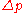the momentum of the wall must be known to this accuracy before the particle passes through. This introduces an uncertainty in the position of the wall and therefore the position of the slit equal to, and if the wall's momentum is known precisely enough to measure the recoil, the slit's position is uncertain enough to disallow a position measurement.

A similar analysis with particles diffracting through multiple slits is given by Richard Feynman
Richard Feynman
Richard Phillips Feynman was an American physicist known for his work in the path integral formulation of quantum mechanics, the theory of quantum electrodynamics and the physics of the superfluidity of supercooled liquid helium, as well as in particle physics...

.

### Einstein's box

Another of Einstein's thought experiments (Einstein's box) was designed to challenge the time/energy uncertainty principle. It is very similar to the slit experiment in space, except here the narrow window the particle passes through is in time:
Consider a box filled with light. The box has a shutter that a clock opens and quickly closes at a precise time, and some of the light escapes. We can set the clock so that the time that the energy escapes is known. To measure the amount of energy that leaves, Einstein proposed weighing the box just after the emission. The missing energy lessens the weight
Mass-energy equivalence
In physics, mass–energy equivalence is the concept that the mass of a body is a measure of its energy content. In this concept, mass is a property of all energy, and energy is a property of all mass, and the two properties are connected by a constant...

of the box. If the box is mounted on a scale, it is naïvely possible to adjust the parameters so that the uncertainty principle is violated.

Bohr spent a day considering this setup, but eventually realized that if the energy of the box is precisely known, the time at which the shutter opens is uncertain. If the case, scale, and box are in a gravitational field then, in some cases, it is the uncertainty of the position of the clock in the gravitational field that alters the ticking rate. This can introduce the right amount of uncertainty. This was ironic as it was Einstein himself who first discovered gravity's effect on time
Gravitational time dilation
Gravitational time dilation is the effect of time passing at different rates in regions of different gravitational potential; the lower the gravitational potential, the more slowly time passes...

.

### EPR paradox for entangled particles

Bohr was compelled to modify his understanding of the uncertainty principle after another thought experiment by Einstein. In 1935, Einstein, Podolsky and Rosen (see EPR paradox
EPR paradox
The EPR paradox is a topic in quantum physics and the philosophy of science concerning the measurement and description of microscopic systems by the methods of quantum physics...

) published an analysis of widely separated entangled
Quantum entanglement
Quantum entanglement occurs when electrons, molecules even as large as "buckyballs", photons, etc., interact physically and then become separated; the type of interaction is such that each resulting member of a pair is properly described by the same quantum mechanical description , which is...

particles. Measuring one particle, Einstein realized, would alter the probability distribution of the other, yet here the other particle could not possibly be disturbed. This example led Bohr to revise his understanding of the principle, concluding that the uncertainty was not caused by a direct interaction.

But Einstein came to much more far-reaching conclusions from the same thought experiment. He believed the "natural basic assumption" that a complete description of reality would have to predict the results of experiments from "locally changing deterministic quantities", and therefore would have to include more information than the maximum possible allowed by the uncertainty principle.

In 1964 John Bell
John Stewart Bell
John Stewart Bell FRS was a British physicist from Northern Ireland , and the originator of Bell's theorem, a significant theorem in quantum physics regarding hidden variable theories.- Early life and work :...

showed that this assumption can be falsified, since it would imply a certain inequality between the probabilities of different experiments. Experimental results confirm the predictions of quantum mechanics, ruling out Einstein's basic assumption that led him to the suggestion of his hidden variables. (Ironically this fact is one of the best pieces of evidence supporting Karl Popper
Karl Popper
Sir Karl Raimund Popper, CH FRS FBA was an Austro-British philosopher and a professor at the London School of Economics...

's philosophy of invalidation of a theory by falsification-experiments. That is to say, here Einstein's "basic assumption" became falsified by experiments based on Bell's inequalities. For the objections of Karl Popper against the Heisenberg inequality itself, see below.)

While it is possible to assume that quantum mechanical predictions are due to nonlocal hidden variables, and in fact David Bohm
David Bohm
David Joseph Bohm FRS was an American-born British quantum physicist who contributed to theoretical physics, philosophy, neuropsychology, and the Manhattan Project.-Youth and college:...

invented such a formulation, this resolution is not satisfactory to the vast majority of physicists. The question of whether a random outcome is predetermined by a nonlocal theory can be philosophical, and it can be potentially intractable. If the hidden variables are not constrained, they could just be a list of random digits that are used to produce the measurement outcomes. To make it sensible, the assumption of nonlocal hidden variables is sometimes augmented by a second assumption — that the size of the observable universe puts a limit on the computations that these variables can do. A nonlocal theory of this sort predicts that a quantum computer
Quantum computer
A quantum computer is a device for computation that makes direct use of quantum mechanical phenomena, such as superposition and entanglement, to perform operations on data. Quantum computers are different from traditional computers based on transistors...

encounters fundamental obstacles when it tries to factor numbers of approximately 10,000 digits or more; an achievable task
Shor's algorithm
Shor's algorithm, named after mathematician Peter Shor, is a quantum algorithm for integer factorization formulated in 1994...

in quantum mechanics.

### Popper's criticism

Karl Popper
Karl Popper
Sir Karl Raimund Popper, CH FRS FBA was an Austro-British philosopher and a professor at the London School of Economics...

approached the problem of indeterminacy as a logician and metaphysical realist
Philosophical realism
Contemporary philosophical realism is the belief that our reality, or some aspect of it, is ontologically independent of our conceptual schemes, linguistic practices, beliefs, etc....

. He disagreed with the application of the uncertainty relations to individual particles rather than to ensembles of identically prepared particles, referring to them as "statistical scatter relations". In this statistical interpretation, a particular measurement may be made to arbitrary precision without invalidating the quantum theory. This directly contrasts the Copenhagen interpretation
Copenhagen interpretation
The Copenhagen interpretation is one of the earliest and most commonly taught interpretations of quantum mechanics. It holds that quantum mechanics does not yield a description of an objective reality but deals only with probabilities of observing, or measuring, various aspects of energy quanta,...

of quantum mechanics, which is non-deterministic
Determinism
Determinism is the general philosophical thesis that states that for everything that happens there are conditions such that, given them, nothing else could happen. There are many versions of this thesis. Each of them rests upon various alleged connections, and interdependencies of things and...

but lacks local hidden variables.

In 1934, Popper published Zur Kritik der Ungenauigkeitsrelationen (Critique of the Uncertainty Relations) in Naturwissenschaften, and in the same year Logik der Forschung
The Logic of Scientific Discovery
The Logic of Scientific Discovery is a 1934 book by Karl Popper. It was originally written in German and titled Logik der Forschung. Then Popper reformulated his book in English and republished it in 1959. This forms the rare case of a major work to appear in two languages, both written and one...

(translated and updated by the author as The Logic of Scientific Discovery in 1959), outlining his arguments for the statistical interpretation. In 1982, he further developed his theory in Quantum theory and the schism in Physics, writing:
[Heisenberg's] formulae are, beyond all doubt, derivable statistical formulae of the quantum theory. But they have been habitually misinterpreted by those quantum theorists who said that these formulae can be interpreted as determining some upper limit to the precision of our measurements.[original emphasis]

Popper proposed an experiment to falsify
Falsifiability
Falsifiability or refutability of an assertion, hypothesis or theory is the logical possibility that it can be contradicted by an observation or the outcome of a physical experiment...

the uncertainty relations, though he later withdrew his initial version after discussions with Weizsäcker
Carl Friedrich von Weizsäcker
Carl Friedrich Freiherr von Weizsäcker was a German physicist and philosopher. He was the longest-living member of the research team which performed nuclear research in Germany during the Second World War, under Werner Heisenberg's leadership...

, Heisenberg
Werner Heisenberg
Werner Karl Heisenberg was a German theoretical physicist who made foundational contributions to quantum mechanics and is best known for asserting the uncertainty principle of quantum theory...

, and Einstein
Albert Einstein
Albert Einstein was a German-born theoretical physicist who developed the theory of general relativity, effecting a revolution in physics. For this achievement, Einstein is often regarded as the father of modern physics and one of the most prolific intellects in human history...

; this experiment may have influenced the formulation of the EPR experiment
EPR paradox
The EPR paradox is a topic in quantum physics and the philosophy of science concerning the measurement and description of microscopic systems by the methods of quantum physics...

. A version of this experiment was realized in 1999.

### Many-worlds uncertainty

The many-worlds interpretation
Many-worlds interpretation
The many-worlds interpretation is an interpretation of quantum mechanics that asserts the objective reality of the universal wavefunction, but denies the actuality of wavefunction collapse. Many-worlds implies that all possible alternative histories and futures are real, each representing an...

originally outlined by Hugh Everett III in 1957 is partly meant to reconcile the differences between the Einstein and Bohr's views by replacing Bohr's wave function collapse with an ensemble of deterministic and independent universes whose distribution is governed by wave functions and the Schrödinger equation
Schrödinger equation
The Schrödinger equation was formulated in 1926 by Austrian physicist Erwin Schrödinger. Used in physics , it is an equation that describes how the quantum state of a physical system changes in time....

. Thus, uncertainty in the many-worlds interpretation follows from each observer within any universe having no knowledge of what goes on in the other universes.

## Matter wave interpretation

According to the de Broglie hypothesis, every object in our Universe is a wave
Wave
In physics, a wave is a disturbance that travels through space and time, accompanied by the transfer of energy.Waves travel and the wave motion transfers energy from one point to another, often with no permanent displacement of the particles of the medium—that is, with little or no associated mass...

, a situation which gives rise to this phenomenon. Consider the measurement of the position of a particle. The particle's wave packet has non-zero amplitude
Amplitude
Amplitude is the magnitude of change in the oscillating variable with each oscillation within an oscillating system. For example, sound waves in air are oscillations in atmospheric pressure and their amplitudes are proportional to the change in pressure during one oscillation...

, meaning that the position is uncertain – it could be almost anywhere along the wave packet. To obtain an accurate reading of position, this wave packet must be 'compressed' as much as possible, meaning it must be made up of increasing numbers of sine waves added together
Superposition principle
In physics and systems theory, the superposition principle , also known as superposition property, states that, for all linear systems, the net response at a given place and time caused by two or more stimuli is the sum of the responses which would have been caused by each stimulus individually...

. The momentum of the particle is proportional to the wavenumber
Wavenumber
In the physical sciences, the wavenumber is a property of a wave, its spatial frequency, that is proportional to the reciprocal of the wavelength. It is also the magnitude of the wave vector...

of one of these waves, but it could be any of them. So a more precise position measurement – by adding together more waves – means that the momentum measurement becomes less precise (and vice versa).

The only kind of wave with a definite position is concentrated at one point, and such a wave has an indefinite wavelength (and therefore an indefinite momentum). Conversely, the only kind of wave with a definite wavelength is an infinite regular periodic oscillation over all space, which has no definite position. So in quantum mechanics, there can be no states that describe a particle with both a definite position and a definite momentum. The more precise the position, the less precise the momentum.

A mathematical statement of the principle is that every quantum state has the property that the root mean square
Root mean square
In mathematics, the root mean square , also known as the quadratic mean, is a statistical measure of the magnitude of a varying quantity. It is especially useful when variates are positive and negative, e.g., sinusoids...

(RMS) deviation of the position from its mean (the standard deviation
Standard deviation
Standard deviation is a widely used measure of variability or diversity used in statistics and probability theory. It shows how much variation or "dispersion" there is from the average...

of the x-distribution):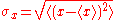times the RMS deviation of the momentum from its mean (the standard deviation of p):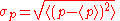can never be smaller than a fixed fraction of Planck's constant: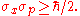The uncertainty principle can be restated in terms of other measurement processes, which involves collapse of the wavefunction. When the position is initially localized by preparation, the wavefunction collapses to a narrow bump in an interval Δx > 0, and the momentum wavefunction becomes spread out. The particle's momentum is left uncertain by an amount inversely proportional to the accuracy of the position measurement:.

If the initial preparation in Δx is understood as an observation or disturbance of the particles then this means that the uncertainty principle is related to the observer effect
Observer effect (physics)
In physics, the term observer effect refers to changes that the act of observation will make on the phenomenon being observed. This is often the result of instruments that, by necessity, alter the state of what they measure in some manner...

. However, this is not true in the case of the measurement process corresponding to the former inequality but only for the latter inequality.

## Additional uncertainty relations

The Heisenberg uncertainty relation and its more formal versions deal explicitly with the quantum operators for position,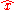and for momentum,. Uncertainty relations for generalized operators have also been derived, such as the Robertson uncertainty relation and for arbitrary Hermitian operators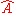and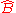is given byA further generalization of the Robertson relation was derived by Schrödinger to give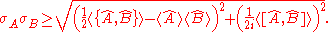Since the Robertson and Schrödinger relations are for general operators, then they can be used to obtain uncertainty relations for any two observables that do not commute. Examples include:
• The kinetic energy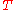and positionof a particle :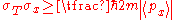• For two orthogonal components of the total angular momentum
Angular momentum
In physics, angular momentum, moment of momentum, or rotational momentum is a conserved vector quantity that can be used to describe the overall state of a physical system...

operator of an object:where i, j, k are distinct and Ji denotes angular momentum along the xi axis. This relation implies that only a single component of a system's angular momentum can be defined with arbitrary precision, normally the component parallel to an external (magnetic or electric) field.

• For the number of electrons in a superconductor and the phase
Phase factor
For any complex number written in polar form , the phase factor is the exponential part, i.e. eiθ. As such, the term "phase factor" is similar to the term phasor, although the former term is more common in quantum mechanics. This phase factor is itself a complex number of absolute value 1...

of its Ginzburg–Landau order parameter### Energy–time uncertainty principle

Other than the position-momentum uncertainty relation, the most important uncertainty relation is that between energy and time. The energy-time uncertainty relation is not, however, an obvious consequence of the general Robertson–Schrödinger relation. Since energy bears the same relation to time as momentum does to space in special relativity
Special relativity
Special relativity is the physical theory of measurement in an inertial frame of reference proposed in 1905 by Albert Einstein in the paper "On the Electrodynamics of Moving Bodies".It generalizes Galileo's...

, it was clear to many early founders, Niels Bohr
Niels Bohr
Niels Henrik David Bohr was a Danish physicist who made foundational contributions to understanding atomic structure and quantum mechanics, for which he received the Nobel Prize in Physics in 1922. Bohr mentored and collaborated with many of the top physicists of the century at his institute in...

among them, that the following relation should hold: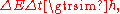but it was not always obvious what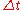precisely meant. The problem is that the time at which the particle has a given state is not an operator belonging to the particle, it is a parameter describing the evolution of the system. As Lev Landau
Lev Landau
Lev Davidovich Landau was a prominent Soviet physicist who made fundamental contributions to many areas of theoretical physics...

once joked "To violate the time-energy uncertainty relation all I have to do is measure the energy very precisely and then look at my watch!"

Nevertheless, Einstein and Bohr understood the heuristic meaning of the principle. A state that only exists for a short time cannot have a definite energy. To have a definite energy, the frequency of the state must accurately be defined, and this requires the state to hang around for many cycles, the reciprocal of the required accuracy.

For example, in spectroscopy, excited states have a finite lifetime. By the time-energy uncertainty principle, they do not have a definite energy, and each time they decay the energy they release is slightly different. The average energy of the outgoing photon has a peak at the theoretical energy of the state, but the distribution has a finite width called the natural linewidth
Spectral linewidth
The spectral linewidth characterizes the width of a spectral line, such as in the electromagnetic emission spectrum of an atom, or the frequency spectrum of an acoustic or electronic system...

. Fast-decaying states have a broad linewidth, while slow decaying states have a narrow linewidth.

The broad linewidth of fast decaying states makes it difficult to accurately measure the energy of the state, and researchers have even used microwave cavities to slow down the decay-rate, to get sharper peaks. The same linewidth effect also makes it difficult to measure the rest mass of fast decaying particles in particle physics
Particle physics
Particle physics is a branch of physics that studies the existence and interactions of particles that are the constituents of what is usually referred to as matter or radiation. In current understanding, particles are excitations of quantum fields and interact following their dynamics...

. The faster the particle decay
Particle decay
Particle decay is the spontaneous process of one elementary particle transforming into other elementary particles. During this process, an elementary particle becomes a different particle with less mass and an intermediate particle such as W boson in muon decay. The intermediate particle then...

s, the less certain is its mass.

One false formulation of the energy-time uncertainty principle says that measuring the energy of a quantum system to an accuracy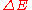requires a time interval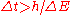. This formulation is similar to the one alluded to in Landau's joke, and was explicitly invalidated by Y. Aharonov
Yakir Aharonov
Yakir Aharonov is an Israeli physicist specializing in quantum physics. He is a Professor of Theoretical Physics and the James J. Farley Professor of Natural Philosophy at Chapman University in California. He is also a distinguished professor in Perimeter Institute.He also serves as a professor...

and D. Bohm
David Bohm
David Joseph Bohm FRS was an American-born British quantum physicist who contributed to theoretical physics, philosophy, neuropsychology, and the Manhattan Project.-Youth and college:...

in 1961 . The timein the uncertainty relation is the time during which the system exists unperturbed, not the time during which the experimental equipment is turned on, whereas the position in the other version of the principle refers to where the particle has some probability to be and not where the observer might look.

Another common misconception is that the energy-time uncertainty principle says that the conservation of energy
Conservation of energy
The nineteenth century law of conservation of energy is a law of physics. It states that the total amount of energy in an isolated system remains constant over time. The total energy is said to be conserved over time...

can be temporarily violated – energy can be "borrowed" from the Universe as long as it is "returned" within a short amount of time. Although this agrees with the spirit of relativistic quantum mechanics, it is based on the false axiom that the energy of the Universe is an exactly known parameter at all times. More accurately, when events transpire at shorter time intervals, there is a greater uncertainty in the energy of these events. Therefore it is not that the conservation of energy is violated when quantum field theory
Quantum field theory
Quantum field theory provides a theoretical framework for constructing quantum mechanical models of systems classically parametrized by an infinite number of dynamical degrees of freedom, that is, fields and many-body systems. It is the natural and quantitative language of particle physics and...

uses temporary electron-positron pairs in its calculations, but that the energy of quantum systems is not known with enough precision to limit their behavior to a single, simple history. Thus the influence of all histories must be incorporated into quantum calculations, including those with much greater or much less energy than the mean of the measured/calculated energy distribution.

In 1932 Dirac offered a precise definition and derivation of the time-energy uncertainty relation in a relativistic quantum theory of "events". But a better-known, more widely used formulation of the time-energy uncertainty principle was given in 1945 by L. I. Mandelshtam and I. E. Tamm
Igor Tamm
Igor Yevgenyevich Tamm was a Soviet physicist and Nobel laureate who received most prestigious Nobel Prize in Physics, jointly with Pavel Alekseyevich Cherenkov and Ilya Frank, for the discovery of Cherenkov radiation, made in 1934.-Biography:Tamm was born in Vladivostok, Russian Empire , in a...

, as follows. For a quantum system in a non-stationary stateand an observable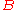represented by a self-adjoint operator, the following formula holds:whereis the standard deviation of the energy operator in the state,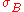stands for the standard deviation of. Although, the second factor in the left-hand side has dimension of time, it is different from the time parameter that enters Schrödinger equation
Schrödinger equation
The Schrödinger equation was formulated in 1926 by Austrian physicist Erwin Schrödinger. Used in physics , it is an equation that describes how the quantum state of a physical system changes in time....

. It is a lifetime of the state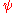with respect to the observable. In other words, this is the time after which the expectation value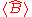changes appreciably.

### Entropic uncertainty principle

While formulating the many-worlds interpretation
Many-worlds interpretation
The many-worlds interpretation is an interpretation of quantum mechanics that asserts the objective reality of the universal wavefunction, but denies the actuality of wavefunction collapse. Many-worlds implies that all possible alternative histories and futures are real, each representing an...

of quantum mechanics in 1957, Hugh Everett III discovered a much stronger formulation of the uncertainty principle. In the inequality of standard deviations, some states, like the wavefunctionhave a large standard deviation of position, but are actually a superposition of a small number of very narrow bumps. In this case, the momentum uncertainty is much larger than the standard deviation inequality would suggest. A better inequality uses the Shannon entropy
Information theory
Information theory is a branch of applied mathematics and electrical engineering involving the quantification of information. Information theory was developed by Claude E. Shannon to find fundamental limits on signal processing operations such as compressing data and on reliably storing and...

of the distribution, a measure of the uncertainty in a random variable described by a probability distributionwhere n is an arbitrary base for the logarithm. The interpretation of Hx is that, for example if n=2, it is the number of bits of information an observer acquires when the value of x is given to accuracyis equal to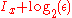. The second part is just the number of bits past the decimal point, the first part is a logarithmic measure of the width of the distribution. For a uniform distribution of width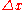the information content is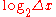bits. This quantity can be negative, which means that the distribution is narrower than one unit, so that learning the first few bits past the decimal point gives no information since they are not uncertain.

Taking the logarithm of Heisenberg's formulation of uncertainty:but the lower bound is not precise.

Everett (and Hirschman) conjectured that for all quantum states (using natural units
Natural units
In physics, natural units are physical units of measurement based only on universal physical constants. For example the elementary charge e is a natural unit of electric charge, or the speed of light c is a natural unit of speed...

):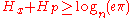This was proven by Beckner in 1975. The equality is attained when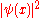is a normalized Gaussian.

## See also

• Canonical commutation relation
Canonical commutation relation
In physics, the canonical commutation relation is the relation between canonical conjugate quantities , for example:[x,p_x] = i\hbar...

• Correspondence principle
Correspondence principle
In physics, the correspondence principle states that the behavior of systems described by the theory of quantum mechanics reproduces classical physics in the limit of large quantum numbers....

• Correspondence rules
Correspondence rules
In quantum mechanics, correspondence rules refers to the principle of replacing physical quantities with operators.Such replacements include energy and momentum, which can be derived informally from taking the time and space derivities of the plane wave function. These show a similarity to the...

• Introduction to quantum mechanics
Introduction to quantum mechanics
Quantum mechanics is the body of scientific principles that explains the behavior of matter and its interactions with energy on the scale of atoms and atomic particles....

• Heisenbug
• Quantum indeterminacy
Quantum indeterminacy
Quantum indeterminacy is the apparent necessary incompleteness in the description of a physical system, that has become one of the characteristics of the standard description of quantum physics...

• The Part and The Whole
The Part and The Whole
The book The Part and the Whole, written by Werner Heisenberg, the German physicist who discovered the uncertainty principle, tells, from his point of view, the history of exploring atomic science and quantum mechanics in the first half of the 20th century.As the subtitle "Talks about atomic...

(book)

## External links

The source of this article is wikipedia, the free encyclopedia.  The text of this article is licensed under the GFDL.